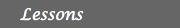MATH EXPLORERS' CLUB Cornell Department of Mathematics

Ehrenfeucht-Fraïssé games

 Introduction1. History of EF games 2. Description 3. Examples 4. FO logic for graphs 5. EF games & FO logic 6. Applications ReferencesDescription of the Ehrenfeucht-Fraïssé games

In the EF game, there are two players, called the Spoiler, and the Duplicator. The board of the game consists of two graphs, let us denote them by A and B. The goal of the Spoiler is to show that the two graphs are different; the goal of the duplicator is to show that they are the same.

The players play a certain number of rounds. Each round consists of the following steps:

• 1. The Spoiler picks either graph A or graph B.
• 2. The selects makes a move. He chooses a vertex from that graph.
• 3. The Duplicator goes next. She responds by picking a vertex from the other graph(the graph that the Spoiler did not select from).
• We denote by xi the vertex selected from A, and by yi the vertex selected from B in this i-th round of the game, regardless of who has selected them.
Let us denote by X = {x1, x2, ... ,xn}, and by Y the set Y = {y1, y2, ... ,yn}.
The Duplicator wins the n rounds EF games on the graphs A and B if the map f from X to Y, f(xi) = yi, i = 1,...,n preserves adjacency and equality. This means that:
• xi, xj are adjacent in A if and only if yi, yj are adjacent in B.
• xi = xj if and only if yi = yj.
In this case, we call the map f a partial isomorphism of the two graphs A and B, and we write A ~n B.
Otherwise the Spoiler wins the n rounds EF game on the graphs A and B.
Intuitively speaking, the Duplicator wins the n rounds game if she can duplicate any n moves that the Spoiler makes. So the n rounds EF game cannot distinguish between A and B. In this case, we write A ~n B. Otherwise the Spoiler wins the n rounds EF game on the graphs A and B.

Observations:

• The Spoiler decides in the beginning of each round which graph to choose from. Its choice may change from round to round.
• If both graphs A and B have more then n vertices, in the n-rounds EF game, there is no point for the Spoiler to repeat his choices. For instance, if in the j-th round, the Spoiler selects a vertex xj equal to a previous xi that he selected in the i-th round, then the Duplicator can repeat her choices as well.
She can simply select yj = yi.
 Top Next lesson